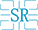# Reflections caused by PCB trace width changes

Release Date：2020-05-12

When PCB routing, it often happens that when the trace passes through an area, due to the limited wiring space in the area, it is necessary to use a thinner line. After passing this area, the line returns to its original width. Changes in trace width will cause impedance changes, so reflection will occur, which will affect the signal. So under what circumstances can this effect be ignored, and under what circumstances must we consider its impact?

# There are three factors related to this effect: the magnitude of the PCB impedance change, the signal rise time, and the delay of the signal on the narrow line.

First discuss the magnitude of the impedance change. Many circuit designs require that the reflected noise be less than 5% of the voltage swing (this is related to the noise budget on the signal). According to the reflection coefficient formula:

ρ = (Z2-Z1) / (Z2 + Z1) = △ Z / (△ Z + 2Z1) ≤5%

The approximate change rate of impedance can be calculated as: △ Z / Z1≤10%

# As you may know, the typical index of impedance on a circuit board is +/- 10%, and the root cause is here.

If the impedance change only occurs once, for example, after the line width changes from 8 mil to 6 mil, the 6 mil width is maintained. To meet the noise budget requirement that the signal reflection noise at the mutation does not exceed 5% of the voltage swing, the impedance change must be less than 10%. This is sometimes difficult to do. Take the microstrip line on the FR4 sheet as an example, let's calculate it. If the line width is 8 mil, the thickness between the line and the reference plane is 4 mil, and the characteristic impedance is 46.5 ohm. After the line width changes to 6mil, the characteristic impedance becomes 54.2 ohms, and the impedance change rate reaches 20%. The amplitude of the reflected signal must exceed the standard. As for how much it affects the signal, it is also related to the signal rise time and the delay of the signal from the driving end to the reflection point. But at least this is a potential problem. Fortunately, the problem can be solved by impedance matching termination.

If the impedance change occurs twice, for example, after the line width changes from 8mil to 6mil, then pulls out 2cm and then returns to 8mil. Then reflection will occur at both ends of the 2cm long 6mil line, once the impedance becomes larger Reflection, then the impedance becomes smaller, and negative reflection occurs. If the interval between two reflections is short enough, the two reflections may cancel each other, thereby reducing the impact. Assuming that the transmission signal is 1V, the first regular reflection has 0.2V reflected, the 1.2V continues to transmit forward, and the second reflection has -0.2 * 1.2 = 0.24v reflected back. Assuming that the length of the 6mil line is extremely short and the two reflections occur almost simultaneously, then the total reflected voltage is only 0.04V, which is less than the noise budget requirement of 5%.

Therefore, whether or not this reflection affects the signal is related to the delay at the impedance change and the signal rise time. Research and experiments have shown that as long as the delay at the impedance change is less than 20% of the signal rise time, the reflected signal will not cause problems. If the signal rise time is 1ns, then the delay at the impedance change is less than 0.2ns corresponding to 1.2 inches, and reflection will not cause problems. In other words, for the case of this example, as long as the length of the 6mil wide trace is less than 3cm, there will be no problem.

When the PCB trace line width changes, it should be carefully analyzed according to the actual situation, whether it causes an impact. There are three parameters to be concerned about: how big is the change in impedance, what is the signal rise time, and how long is the neck-shaped part of the line width change. Approximately estimate according to the above method, and leave a certain margin appropriately. If possible, minimize the length of the neck.

It should be pointed out that in the actual PCB processing, the parameters cannot be as accurate as the theory. The theory can provide guidance for our design, but it cannot be copied, and it cannot be dogmatic. After all, this is a practical science. The estimated value should be appropriately revised according to the actual situation, and then applied to the design.

## Service hotline：

(E-Mail): inquiry@szstariver.com

(Tel): +86-139-029-12758 (Tiger Huang）

(Fax): 0755-81499249

Add :  Haosi West industrial zone,Sha jing town Bao an district Shenzhen

PCB
Capacity
Main Application
Quality System
Factory
Production Process